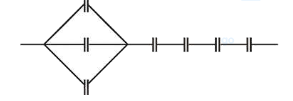# Seven capacitors, each of capacitanceQuestion:

Seven capacitors, each of capacitance $2 \mu \mathrm{F}$, are to be connected in a configuration to obtain an effective

capacitance of $\left(\frac{6}{13}\right) \mu \mathrm{F}$. Which of the combinations, shown

in figures below, will achieve the desired value?

1.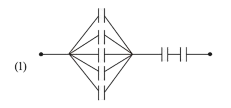2.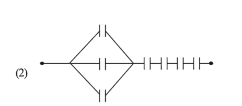3. (3)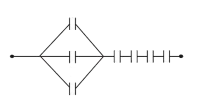4. (4)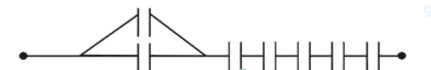Correct Option: , 2

Solution:

(2) As required equivalent capacitance should bo

$\mathrm{C}_{e q}=\frac{6}{13} \mu \mathrm{F}$

Therefore three capacitors must be in parallel and 4 must be in series with it.

$\frac{1}{C_{\infty}}=\left[\frac{1}{3 C}\right]+\left[\frac{1}{C}+\frac{1}{C}+\frac{1}{C}+\frac{1}{C}\right]$

$\mathrm{C}_{\text {eq }}=\frac{3 \mathrm{C}}{13}=\frac{6}{13} \mu \mathrm{F} \quad[$ as $\mathrm{C}=2 \mu \mathrm{F}]$

So, desired combination will be as below:\